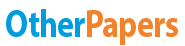# Ust Inc Case Study

Essay by   •  August 24, 2011  •  Study Guide  •  326 Words (2 Pages)  •  3,073 Views

## Essay Preview: Ust Inc Case Study

Report this essay
Page 1 of 2

3. Should UST Inc. undertake the \$1 billion recapitalization? Calculate the marginal (or incremental)

effect on UST's value, assuming that the entire recapitalization is implemented immediately

(January 1, 1999).

(a)Assume a 38% tax rate.

(b)Prepare a pro-forma income statement to analyze whether UST will be able to make interest

payments.

(c)For the basic analysis, assume the \$1 billion in new debt is constant and perpetual.

Should UST alter the new debt via a different level or a change in the amount of debt through time?

a) UST should undertake the \$1 billion recapitalization.

The marginal effect on UST's value of implementing the recapitalization, assuming

that the plan is implemented immediately on January 1, 1999, is equal to the sum of

the tax shield minus the present value of financial distress.

VL = VU + Tax Shield  Expected Bankruptcy Cost

à VL = VU + tD - PV (Financial Distress)

à VL = VU + tD - (probability of bankruptcy * cost of bankruptcy)

à VL = VU + tD - (probability of bankruptcy * (g * VU))

Where g is some constant that is usually valued between 5% and 30%

Therefore, the marginal effect is:

?VL = tD - (probability of bankruptcy * (g * VU))

= (0.38)(\$1billion) - (0.0028)(0.30)(\$6.5billion)

= \$0.375 billion

Notes:

(1) The probability of bankruptcy is inferred using an AAA credit rating (from lecture

notes). See spreadsheet, row EBIT interest coverage ratio. The ratio after

recapitalization falls into the AAA rank which has a ratio of 12.9.

(2) g, or the constant fraction of VU that we lose in event of bankruptcy, is given a

conservative estimate of 0.30 for conservative reasons.

(3) VU, or the value of the unlevered firm, is obtained by summing USTs market

capitalization (\$6.4billion) and long-term debt (\$0.1billion) in 1998. Although the

firm is technically levered (D/V = 1.5%)

...

...